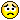#Message Boards

0
|
4890 Views
|
12 Replies
|
0 Total Likes
View groups...
Share
GROUPS:

Posted 10 years ago
 I have to solve the task about mathematical pendulum.And I have formula:x''(t)+g/k*sin(x(t))=0, where x - angle of pendulum.And for solve this we have x(t=0) = 0, x'(t=0) = aBut when I write in mathematica:DSolve[{x''[t] + g/l*Sin[x[t]] == 0, x' == 0, x == a}, x[t], t]I have three errors: Solve::ifun: Inverse functions are being used by Solve, so some solutions may not be found; use Reduce for complete solution information. >>DSolve::bvfail: For some branches of the general solution, unable to solve the conditions. >>DSolve::bvfail: For some branches of the general solution, unable to solve the conditions. >>I have spend several hours, but with no result...Please, help me to understand - what happens...
12 Replies
Sort By:
Posted 10 years ago
 Another approach, if you're using NDSolve repeatedly, and only changing one or more parameters each time, is to use ParametricNDSolve, to set up the problem.That avoid repeating the setup each time.
Posted 10 years ago
 Thank you for this tip Frank, I suspected that there exists more right way).I doesn't fully understand this single string, but it shows me ways, where I can find gold in futurePosted 10 years ago
 Rather than running NDSolve multiple times, you can convert the problem into one PDE.sln = NDSolve[{D[x[t, a], {t, 2}] == -Sin[x[t, a]],    Derivative[1, 0][x][0, a] == 0, x[0, a] ==  a},   x, {t, 0, 12}, {a, 0, \[Pi]/2}]
Posted 10 years ago
 We have done it!)We have to plot dependence between period and starting angle.And we have done it : tt = {};  ti = {}; Do[f = x[t] /. NDSolve[{x''[t] + g/l*Sin[x[t]] == 0, x' == 0, x == i}, x[t], {t, 0, 12}][];    m1 = t /. FindMinimum[f, {t, 0, 12}][];    per = 2*m1;    AppendTo[tt, per];     AppendTo[ti, i],    {i, 0.1, Pi/2 - 0.1, 0.1}]ListPlot[{tt}, DataRange -> {0.1, Pi/2 - 0.1}]Thank you very much - without your help this task would be un..resolvable)
Posted 10 years ago
 I should have mentioned that my previous post was done on my Raspberry Pi, so it is actually Version 10.  Version 9 returns no result.
Posted 10 years ago
 DSolve[{x''[t] + Sin[x[t]] == 0, x' == 0, x == 1}, x[t], t]{{x[t] ->    2 JacobiAmplitude[     1/2 (-t Sqrt[2 (1 - Cos)] + 2 EllipticF[1/2, Csc[1/2]^2]),      Csc[1/2]^2]}}
Posted 10 years ago
 May be I need to Clear 'a' and to add it as variable in NDSolve?Something like this: g = 1; l = 1; f = x[t] /. NDSolve[{x''[t] + g/l*Sin[x[t]] == 0, x' == 0, x == a}, x[t], {t, 0, 12}, {a, 0, Pi/2}][];Plot3D[f, {t, 0, 12}, {a, 0, Pi/2}]But this code doesn't workPosted 10 years ago
 The analytic solution to the pendulum problem involves elliptic integrals:http://en.wikipedia.org/wiki/Pendulum_%28mathematics%29
Posted 10 years ago
 May be there are more common way to get period?
Posted 10 years ago
 Thank you very much!It works!)But one step further and we stopped again((Our next step - to find dependence period from starting angle (a).Period we can find :g = 1; l = 1; a = 1;f = x[t] /. NDSolve[{x''[t] + g/l*Sin[x[t]] == 0, x' == 0, x == a},  x[t], {t, 0, 12}][]; mt = (t /. FindMinimum[f, {t, 8, 12}][]) - (t /.    FindMinimum[f, {t, 0, 6}][])But what I need to do then... I cannot understand...So I whant to find mt - could you please to help me?sorry for my english... it is not my organic language an I am under time pressure...
Posted 10 years ago
 This takes what you have already written and makes a few very small changes.Will this provide the solution that you need?NDSolve requires that all parameters be given constant numeric values.In:= g=1; l=1; a=1;f = x[t]/.NDSolve[{x''[t] + g/l*Sin[x[t]]==0, x'==0, x==a}, x[t], {t, 0, 8}][];Plot[f, {t, 0, 8}]Out= ...PlotSnipped...
Posted 10 years ago
 Hello and welcome to the Wolfram Community! Your code is missing some parts. Please take a few minutes to read this tutorial about correct posting  especially of Mathematica code:How to type up a post: editor tutorial & general tipsIf you will not follow the tutorial, other members of community may not be able to test your code. To edit your post  click Edit in the lower right corner of your post.